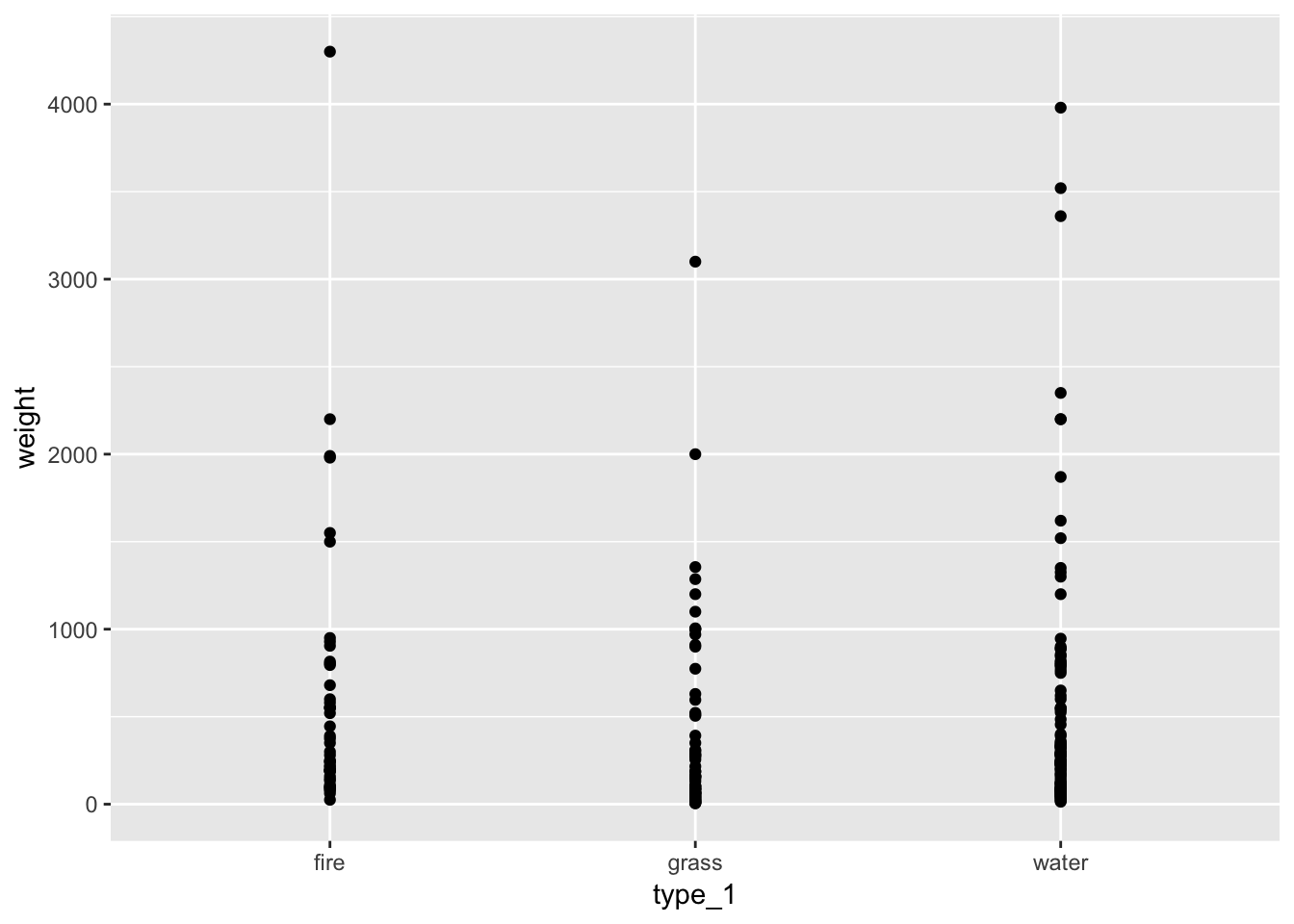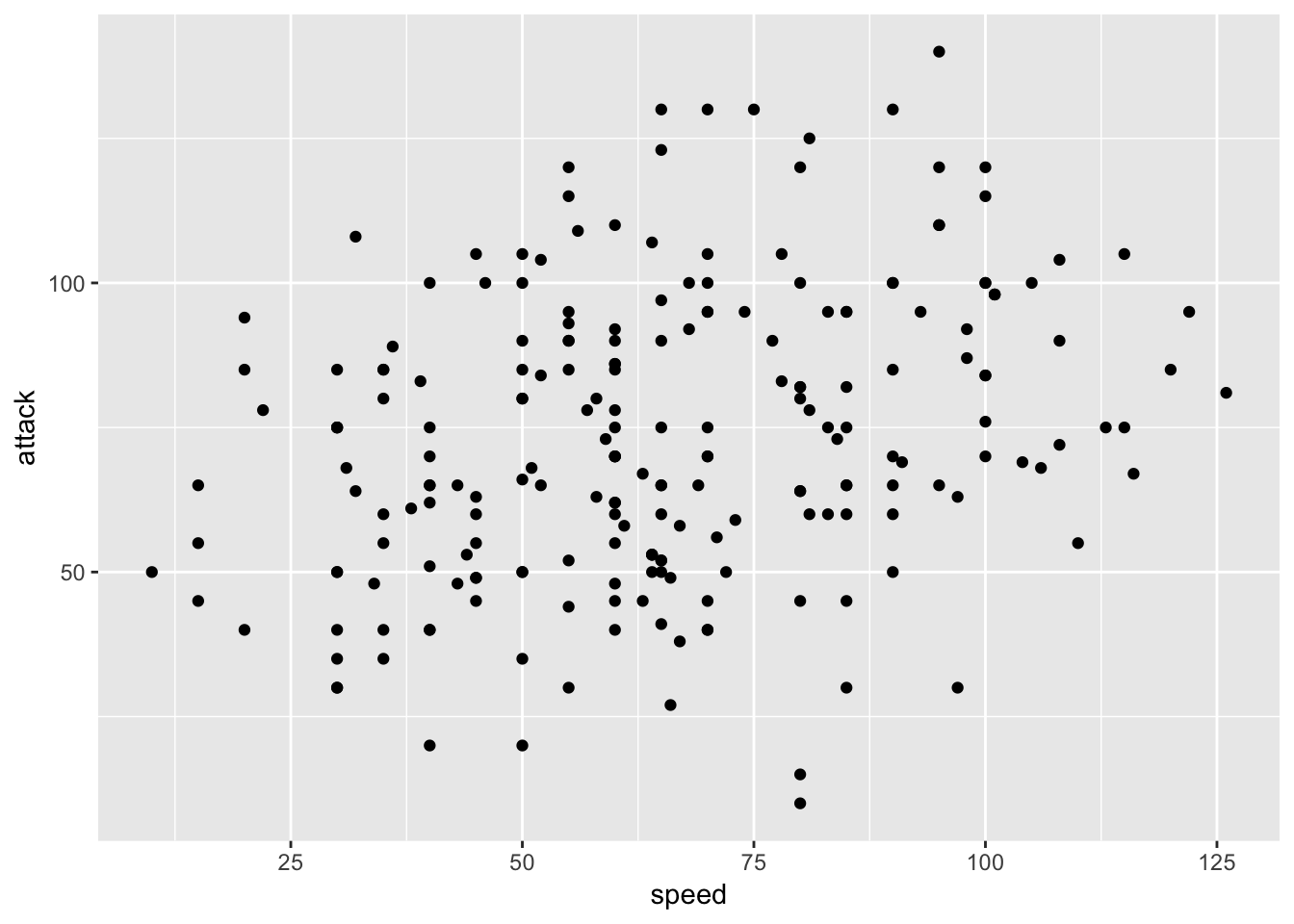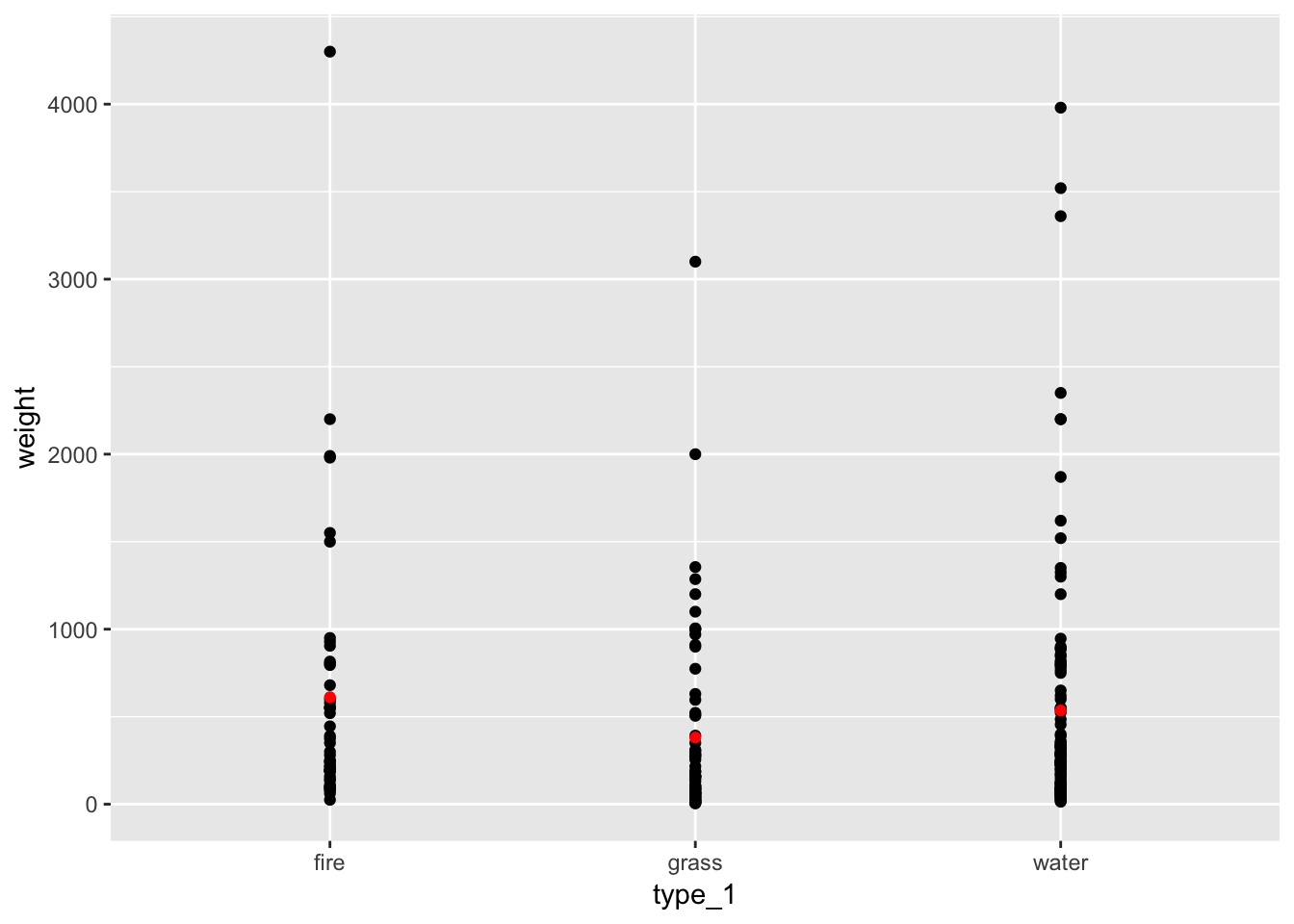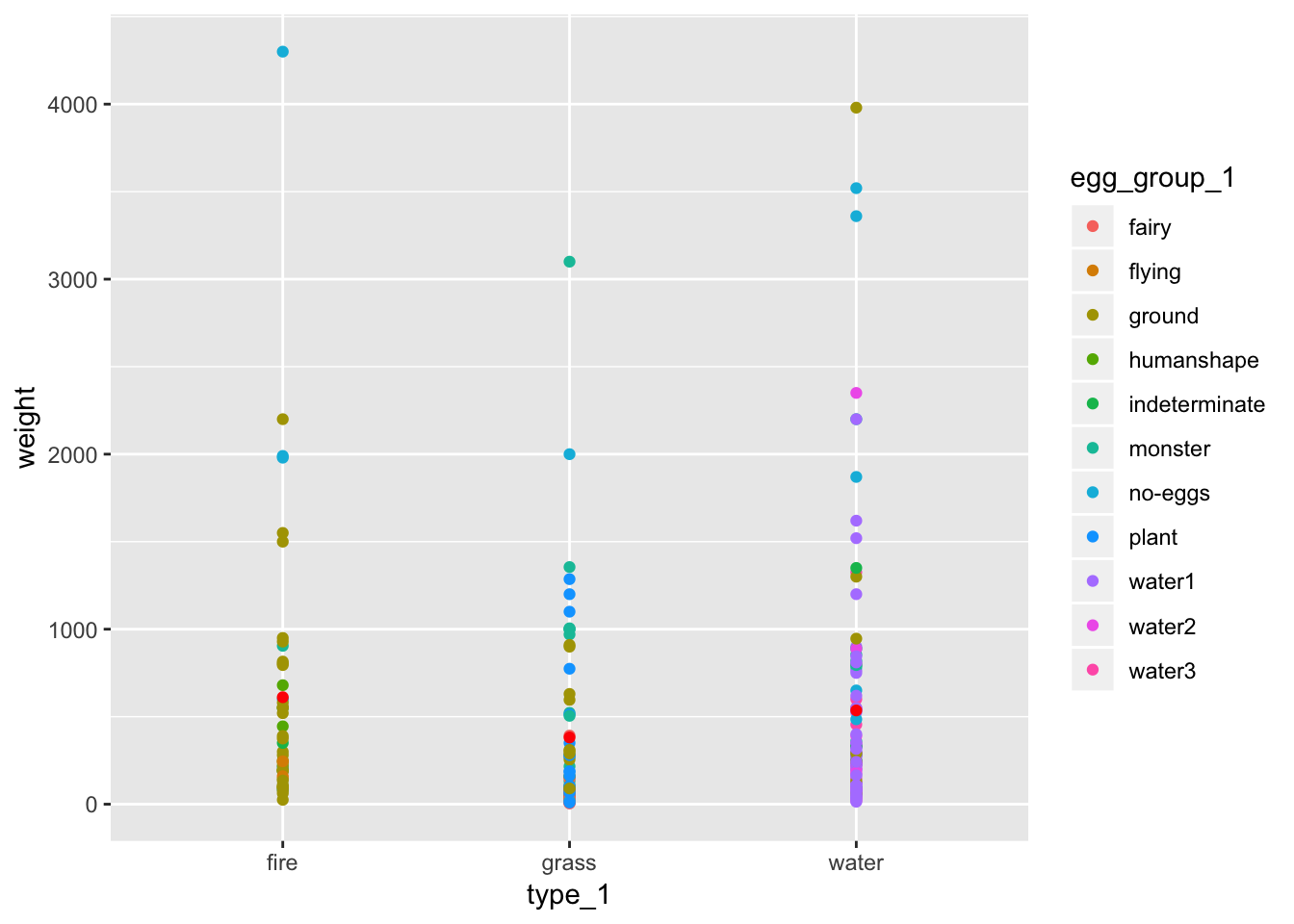# Writing efficient and streamlined R code with help from the new `rlang` package

The powerful new rlang package developed by Lionel Henry and Hadley Wickham at RStudio gives R programmers new tools to control evaluation of variables, compose formulas and functions and modify environments. R programmers can tackle these tasks without `rlang`, but the package gives us more straightforward approaches and solutions.

Although broadly useful, to a certain extent `rlang` provides tools for tool builders rather than tools for casual users. In `rlang`, for example, you might use `!!` or even `!!!` to perform tasks. These are clearly not as easily understood out of the box as R functions with clear names such as `filter()` and `arrange()`.

But what `rlang` adds in opaque syntax the package makes up for in powerful and concise tools. Of particular value, are `rlang's` tools for evaluating quoted and un-quoted variables in different contexts. For example, base R often makes use of strings as variable names as in `dat[,"variable1"]`. More recent tools, like those in the `dplyr` package, tend to use bare variable names such as `select(dat, variable1)`. As you write your own functions `rlang` helps you make use of both strategies and to move between them — evaluating strings within functions that use bare-name syntax and vice-versa.

Popular R packages like `dplyr` and `ggplot2` now use `rlang` to power the bare name syntax found in key functions such as `select()`, `mutate()` or `aes()` and `rlang` easily allows you to use the same strategies in your own R code.

## Using `ggplot2` to demonstrate the power of `rlang`

To illustrate the power of `rlang` we will build a custom plotting function with the brand new version of `ggplot2` (version 3.0.0). The function will take a data frame and two column names and plot the two variables as a dot chart. In the final step we will bring in the `dplyr` package to help us include means on the plot.

In each example we are going to use a slice of the `pokemon` data set from the `highcharter` package.

``````library(dplyr)
# install.packages("highcharter")
data(pokemon, package = "highcharter")

# limit our data to water, grass, and fire types
pokemini <- pokemon[pokemon\$type_1 %in% c("water", "grass", "fire"), ]
glimpse(pokemini)``````
``````## Observations: 217
## Variables: 20
## \$ id              <dbl> 1, 2, 3, 4, 5, 6, 7, 8, 9, 37, 38, 43, 44, 45,...
## \$ pokemon         <chr> "bulbasaur", "ivysaur", "venusaur", "charmande...
## \$ species_id      <int> 1, 2, 3, 4, 5, 6, 7, 8, 9, 37, 38, 43, 44, 45,...
## \$ height          <int> 7, 10, 20, 6, 11, 17, 5, 10, 16, 6, 11, 5, 8, ...
## \$ weight          <int> 69, 130, 1000, 85, 190, 905, 90, 225, 855, 99,...
## \$ base_experience <int> 64, 142, 236, 62, 142, 240, 63, 142, 239, 60, ...
## \$ type_1          <chr> "grass", "grass", "grass", "fire", "fire", "fi...
## \$ type_2          <chr> "poison", "poison", "poison", NA, NA, "flying"...
## \$ attack          <int> 49, 62, 82, 52, 64, 84, 48, 63, 83, 41, 76, 50...
## \$ defense         <int> 49, 63, 83, 43, 58, 78, 65, 80, 100, 40, 75, 5...
## \$ hp              <int> 45, 60, 80, 39, 58, 78, 44, 59, 79, 38, 73, 45...
## \$ special_attack  <int> 65, 80, 100, 60, 80, 109, 50, 65, 85, 50, 81, ...
## \$ special_defense <int> 65, 80, 100, 50, 65, 85, 64, 80, 105, 65, 100,...
## \$ speed           <int> 45, 60, 80, 65, 80, 100, 43, 58, 78, 65, 100, ...
## \$ color_1         <chr> "#78C850", "#78C850", "#78C850", "#F08030", "#...
## \$ color_2         <chr> "#A040A0", "#A040A0", "#A040A0", NA, NA, "#A89...
## \$ color_f         <chr> "#81A763", "#81A763", "#81A763", "#F08030", "#...
## \$ egg_group_1     <chr> "monster", "monster", "monster", "monster", "m...
## \$ egg_group_2     <chr> "plant", "plant", "plant", "dragon", "dragon",...
## \$ url_image       <chr> "1.png", "2.png", "3.png", "4.png", "5.png", "...``````

## A first go

Create a `plot_points1()` function and try calling the function with bare name arguments.

``````# Error ahead!
library(ggplot2)

plot_points1 <- function(.data, x, y) {
ggplot(.data, aes(x, y)) +
geom_point()
}

# Feed the function bare variable names
plot_points1(pokemini, type_1, weight)``````
``## Error in FUN(X[[i]], ...): object 'type_1' not found``

But you’ll notice that running the example gives an error. The name we provided as argument one, `type_1`, is evaluated prematurely in the wrong context. In the past we might have used `aes_string()` instead of `aes()` and asked the user to provide string inputs (i.e. `plot_points1(pokemini, "type_1", "weight"`). But this is inconsistent with `ggplot2` behavior and `aes_string()` is now soft-deprecated in favor of the following strategy. We want our plotting function to work without `aes_string()` and with bare names.

## It lives!

To alter `plot_points1()` to allow bare name inputs we will use two new tools provided by `rlang`, `enquo()` and `!!` (referred to as “bang bang”). `enquo()` is a means of preserving an argument for later evaluation. The `!!` operator is the compliment and allows us to evaluate a captured value.

``````plot_points2 <- function(.data, x, y) {
x <- enquo(x)
y <- enquo(y)
ggplot(.data, aes(!!x, !!y)) +
geom_point()
}
plot_points2(pokemini, type_1, weight)
``````Cool! But, let’s take a moment to break down what contributed to our success.

``````x <- enquo(x)
y <- enquo(y)``````

Our function begins by capturing the arguments `x` and `y`. This is critical. Think about handing a Lego set to a friend. Instinctively they use the default instructions to build the set. What if instead we handed them a Lego set and a different booklet of instructions? Now they can build an entirely different creation with the same Legos. That is the power of `enquo()`. Before calling `x <- enquo(x)` R had its own plans for evaluating `x`, but now we have control over the instructions for evaluating `x`. In our case, we need to evaluate the arguments `x` and `y` inside `aes()`.

``aes(!!x, !!y)``

In our call to `aes()` we can force evaluation of the captured `x` and `y` using `!!`. Jumping back to Legos, this is us telling our friend to build using the new instructions. Within the proper context, the data passed to `ggplot()`, our values stored in `x` and `y`, `type_1` and `weight` respectively, are understood, no more error.

For good measure let’s try another combination of variables.

``plot_points2(pokemini, speed, attack)``Calculating and plotting averages

Let’s add yet another feature to our `plot_points()` function. We will include mean values in our plot. Fortunately for us `dplyr`’s verbs support `!!`, so with our new knowledge of `!!` and `enquo()` we can compute averages on the fly. Take a look at the final product followed by a breakdown of the code.

``````plot_points3 <- function(.data, x, y) {
x <- enquo(x)
y <- enquo(y)
averages <- .data %>%
group_by(!!x) %>%
summarise(avg_y = mean(!!y, na.rm = TRUE))

ggplot() +
geom_point(data = .data, aes(!!x, !!y)) +
geom_point(data = averages, aes(!!x, avg_y), color = "red")
}``````

Our new function computes averages for our `y` variable and adds an additional set of points to our plot. Let’s take a look at the new chunk of code,

``````averages <- .data %>%
group_by(!!x) %>%
summarise(avg_y = mean(!!y, na.rm = TRUE))``````

In this snippet we limit our data to the two relevant variables, group the data by our x variable, and compute mean values for each group of y values.

``group_by(!!x)``

As in `aes()` and `select()` we use `!!` to evaluate `x`. Remember, we captured `x` with `enquo()`. At this point our data is grouped by `type_1`.

``summarise(avg_y = mean(!!y, na.rm = TRUE))``

In our final step we evaluate `y`, `!!y`, and pass the column to `mean()`. With that we have calculated our mean values. Let’s try out the function.

``plot_points3(pokemini, type_1, weight)``A final transmogrification

As our first walk through rlang concludes, let’s look at one last version of the `plot_points()` function. In this version any number of additional named arguments are passed on to `aes()`. This, for example, would allow us to color points by another variable `egg_group_1`. This is done with the help of the ellipsis, `...`, which allows a function to accept any number of additional arguments.

``````plot_points4 <- function(.data, x, y, ...) {
x <- enquo(x)
y <- enquo(y)
args <- enquos(...)

averages <- .data %>%
group_by(!!x) %>%
summarise(avg_y = mean(!!y, na.rm = TRUE))

ggplot(.data, aes(!!x, !!y, !!!args)) +
geom_point() +
geom_point(mapping = aes(!!x, avg_y), data = averages,color = "red")
}
plot_points4(pokemini, type_1, weight, color = egg_group_1)
``````To learn more about `enquo()`, `!!`, and friends checkout the rlang package, the dplyr vignette on programming with dplyr, and upcoming posts right here!

Posted in R

## One response

1.John Mount says:

Neat. I suggest readers also give our wrapr-based solution a try:

library("ggplot2")
library("dplyr")
library("wrapr")
data(pokemon, package = "highcharter")
pokemini <- pokemon[pokemon\$type_1 %in% qc(water, grass, fire), , drop = FALSE]

plot_pointsw <- function(.data, x, y, color) c(
X = x,
Y = y,
COLOR = color
) %in_block% {
averages <- .data %>%
group_by(X) %>%
summarise(avg_y = mean(Y, na.rm = TRUE))

ggplot(.data, aes(X, Y, color = COLOR)) +
geom_point() +
geom_point(mapping = aes(X, avg_y), data = averages, color = "red")
}

plot_pointsw(pokemini, "type_1", "weight", "egg_group_1")